# SLAM Beginner's Program ~Fundamental knowledge~

## Introduction

I studied SLAM refer to the following book. I'm introducing my studying log in dividing into multiple articles. In this article, I wrote some fundamental knowledge of SLAM as memo.

## Executable form

First problem of executing SLAM is how a trajectory for getting sensor data should be decided. There are 2 kinds of way to decide the trajectory.

• A robot is given the trajectory by human
• The robot decide the trajectory automatically

## Type

SLAM type Dimension of robot pos Dimension of map Sensor type
2D/2D type 2D 2D 2D LiDAR, Odometry, Gyro
2D/3D type 2D 3D 2D/3D LiDAR, Camera, Odometry, Gyro
3D/3D type 3D 3D 3D LiDAR, Camera, IMU

## Diagram of 2D SLAM

The following diagram is an example of 2D/2D SLAM.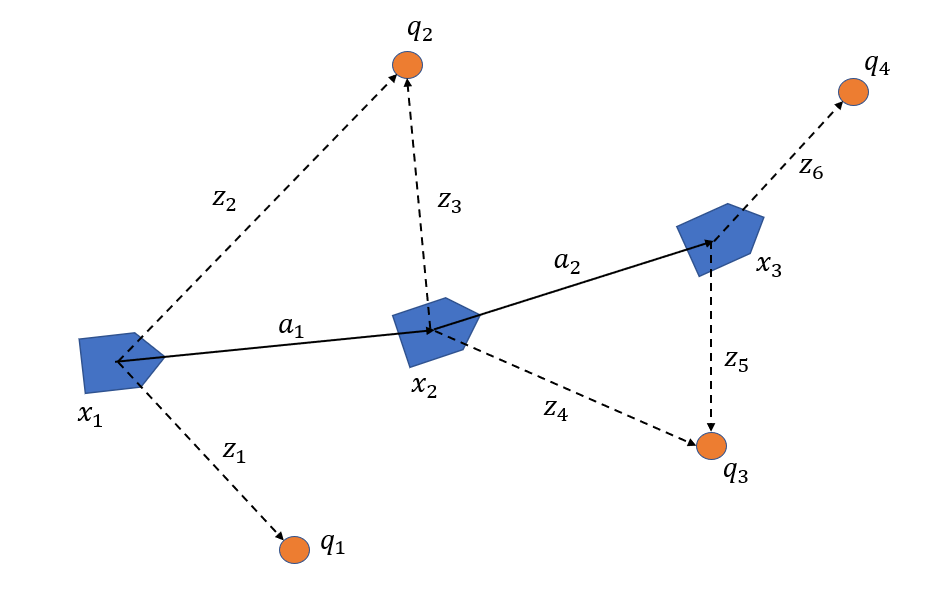• State of Robot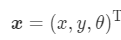• Measurement data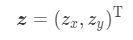• Landmark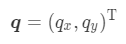• Amount of movement by Odometry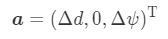• Rotation matrix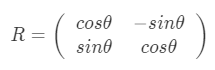• Translation vector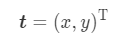• Calculating landmark position depend on robot position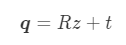• Predicting robot position by odometry
The amount of movement by odometry is minute and the robot can be assumed that it is moving straight. At the same time, an component y can be assumed as 0.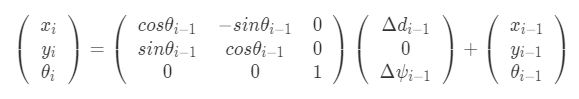This calculation can be expressed like the following formula.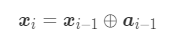## Accumulated error problem

To resolve this problem, the robot need to measure same landmark multiple times. And then, the robot position can be modified by calculating backward with measured landmark position. In the above diagram, the following simultaneous equations. x1 is an initial position and can not be decided. This is defined as an appropriate constant valuables(usually, the origin of map coordinate system).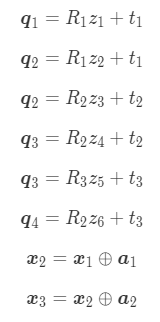Typically, this simultaneous equations can be solved by least squares method.

## Data association

Typically, association with only position constraint is not efficient. If there were two landmarks at almost same position, it would be difficult to decide which landmarks should be associated. Firstly, association candidates are reduced with an feature value. After that, position constraint will be used for the association.

## Loop closure

Loop closure is executed by the following sequence.

• Loop detection
Detecting what the robot returned same position. The following diagram is before loop detection. Land markand,andare same landmarks each other. At that time, the robot positionwill be modified as a position whereand,andbecome same.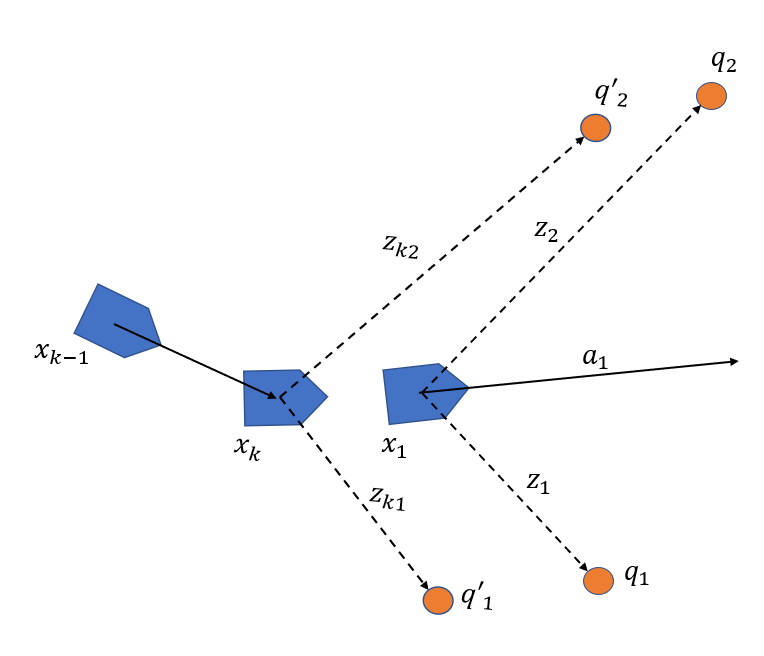• Modifying robot trajectory and map
The original robot positionwill be modified as follow. And then,need to be modified too because the difference of those positions.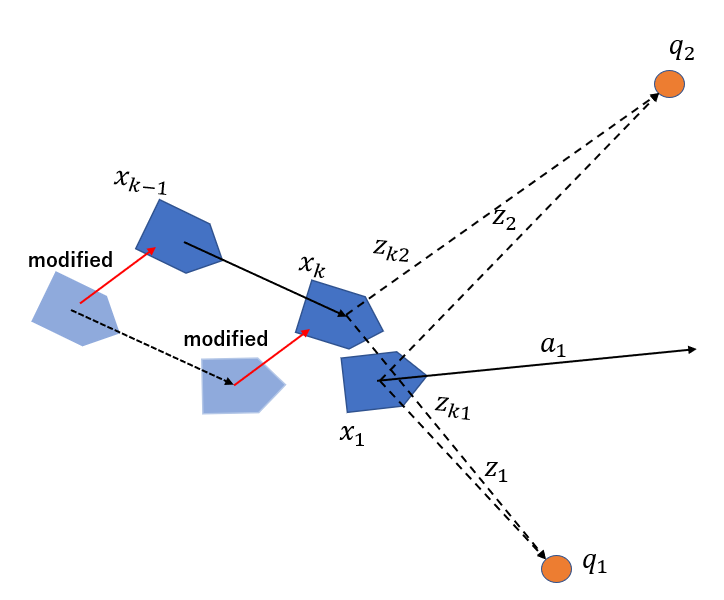## Batch processing

• Robot needs sufficient many sensor data to execute SLAM. This processing is not suitable for real time processing.
• It takes long time to process but an accuracy of map is good.

## Sequential processing

• Map is being constructing in real time sequentially. This is effective to work in a unknown or dynamic environment.
• Only part of all sensor data is used. The processing time is short but an accuracy of map is not good.